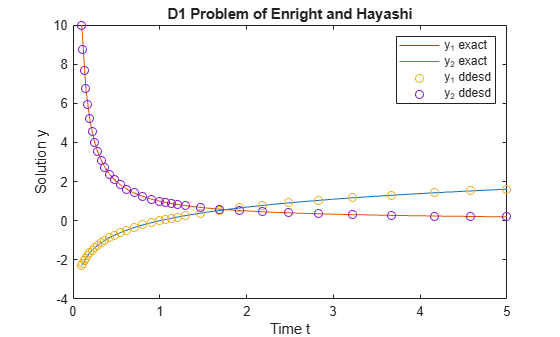DDE with State-Dependent Delays

This example shows how to use ddesd to solve a system of DDEs (delay differential equations) with state-dependent delays. This system of DDEs was used as a test problem by Enright and Hayashi .

The system of equations is

${{\mathit{y}}_{1}}^{\prime }\left(\mathit{t}\right)={\mathit{y}}_{2}\left(\mathit{t}\right),$

${{\mathit{y}}_{2}}^{\prime }\left(\mathit{t}\right)=-{\mathit{y}}_{2}\left({\mathit{e}}^{1-{\mathit{y}}_{2}\left(\mathit{t}\right)}\right)\cdot {\mathit{y}}_{2}{\left(\mathit{t}\right)}^{2}\cdot {\mathit{e}}^{1-{\mathit{y}}_{2}\left(\mathit{t}\right)}.$

The history functions for $\mathit{t}\le 0.1$ are the analytical solutions

${\mathit{y}}_{1}\left(\mathit{t}\right)=\mathrm{log}\left(\mathit{t}\right),$

${\mathit{y}}_{2}\left(\mathit{t}\right)=\frac{1}{\mathit{t}}.$

The time delays in the equations are only present in $\mathit{y}$ terms. The delays depend only on the state of the second component ${\mathit{y}}_{2}\left(\mathit{t}\right)$, so the equations form a system of state-dependent delay equations.

To solve this system of equations in MATLAB, you need to code the equations, delays, and history before calling the delay differential equation solver ddesd, which is meant for systems with state-dependent delays. You either can include the required functions as local functions at the end of a file (as done here), or save them as separate, named files in a directory on the MATLAB path.

Code Delays

First, write a function to define the time delays in the system. The only delay present in this system of equations is in the term$-{\mathit{y}}_{2}\left({\mathit{e}}^{1-{\mathit{y}}_{2}\left(\mathit{t}\right)}\right)$.

function d = dely(t,y)
d = exp(1 - y(2));
end

Note: All functions are included as local functions at the end of the example.

Code Equation

Now, create a function to code the equations. This function should have the signature dydt = ddefun(t,y,Z), where:

• t is time (independent variable).

• y is the solution (dependent variable).

• Z(n,j) approximates the delays ${\mathit{y}}_{\mathit{n}}\left(\mathit{d}\left(\mathit{j}\right)\right)$, where the delay $\mathit{d}\left(\mathit{j}\right)$ is given by component j of dely(t,y).

These inputs are automatically passed to the function by the solver, but the variable names determine how you code the equations. In this case:

• Z(2,1)$\text{\hspace{0.17em}}\to {\mathit{y}}_{2}\left({\mathit{e}}^{1-{\mathit{y}}_{2}\left(\mathit{t}\right)}\right)$

function dydt = ddefun(t,y,Z)
dydt = [y(2);
-Z(2,1)*y(2)^2*exp(1 - y(2))];
end

Code Solution History

Next, create a function to define the solution history. The solution history is the solution for times $\mathit{t}\le {\mathit{t}}_{0}$.

function v = history(t) % history function for t < t0
v = [log(t);
1./t];
end

Solve Equation

Finally, define the interval of integration $\left[{\mathit{t}}_{0}\text{\hspace{0.17em}\hspace{0.17em}}{\mathit{t}}_{\mathit{f}}\right]$ and solve the DDE using the ddesd solver.

tspan = [0.1 5];
sol = ddesd(@ddefun, @dely, @history, tspan);

Plot Solution

The solution structure sol has the fields sol.x and sol.y that contain the internal time steps taken by the solver and corresponding solutions at those times. (If you need the solution at specific points, you can use deval to evaluate the solution at the specific points.)

Plot the two solution components against time using the history function to calculate the analytical solution within the integration interval for comparison.

ta = linspace(0.1,5);
ya = history(ta);

plot(ta,ya,sol.x,sol.y,'o')
legend('y_1 exact','y_2 exact','y_1 ddesd','y_2 ddesd')
xlabel('Time t')
ylabel('Solution y')
title('D1 Problem of Enright and Hayashi')Local Functions

Listed here are the local helper functions that the DDE solver ddesd calls to calculate the solution. Alternatively, you can save these functions as their own files in a directory on the MATLAB path.

function dydt = ddefun(t,y,Z) % equation being solved
dydt = [y(2);
-Z(2,1).*y(2)^2.*exp(1 - y(2))];
end
%-------------------------------------------
function d = dely(t,y) % delay for y
d = exp(1 - y(2));
end
%-------------------------------------------
function v = history(t) % history function for t < t0
v = [log(t);
1./t];
end
%-------------------------------------------

References

 Enright, W.H. and H. Hayashi. “The Evaluation of Numerical Software for Delay Differential Equations.” In Proceedings of the IFIP TC2/WG2.5 working conference on Quality of numerical software: assessment and enhancement. (R.F. Boisvert, ed.). London, UK: Chapman & Hall, Ltd., pp. 179-193.